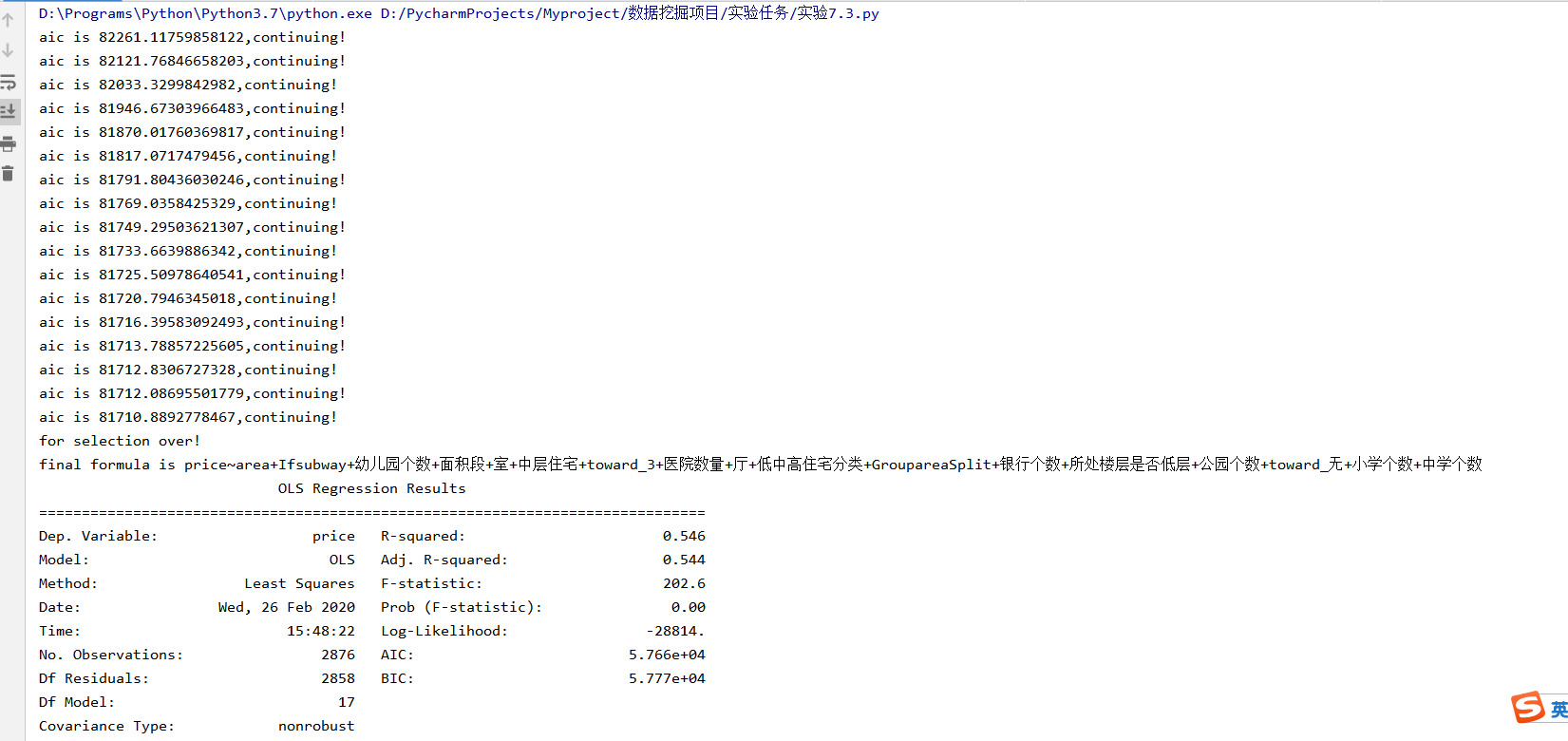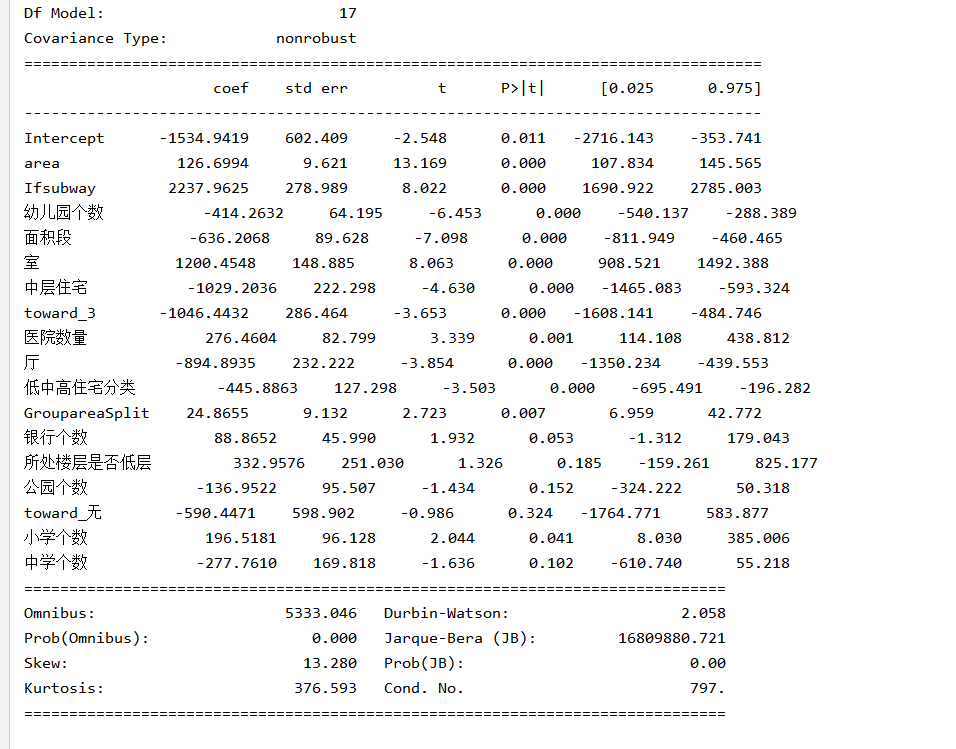# 【python 用逐步回归筛选变量】10分钟快速了解逐步回归筛选变量

1.Forwardselection：从前向后，首先模型中只有一个对因变量影响最显著的自变量，之后尝试将加入另一自变量，观察加入自变量后的整个模型对因变量的影响是否显著增加(这里需要进行检验，如F,t,r方),通过这一过程反复迭代，直到没有自变量再符合加入模型的条件；

2.Backwardelimination：从后向前，此时，所有变量均放入模型，之后尝试将其中一个自变量从模型中剔除，看整个模型对因变量的影响是否有显著变化，之后将使影响减少的最小的变量剔除出队列，此过程不断迭代，直到没有自变量符合剔除的条件。

3.向前向后逐步回归：即前两种的结合，不是一味的增加变量，而是增加一个后，对整个模型的所有变量进行检验，剔除作用不显著的变量，最终得到一个相对最优的变量组合。


# -*- coding: utf-8 -*-

# 载入包
import pandas as pd
from statsmodels.formula.api import ols #加载ols模型
from sklearn.preprocessing import LabelEncoder

# 读取数据
data_path='D:/机器学习/实验作业/实验7/'

# 清洗面积段这个标签
le = LabelEncoder()
y6 = le.fit_transform(data['面积段'])
data['面积段']=y6

house_data=data

#分训练集测试集
import random
random.seed(123) #设立随机数种子
a=random.sample(range(len(house_data)),round(len(house_data)*0.3))
house_test=[]
for i in a:
house_test.append(house_data.iloc[i])
house_test=pd.DataFrame(house_test)
house_train=house_data.drop(a)

# print(house_train)
# print(house_test)

#定义向前逐步回归函数
def forward_select(data,target):
variate=set(data.columns)  #将字段名转换成字典类型
variate.remove(target)  #去掉因变量的字段名
selected=[]
current_score,best_new_score=float('inf'),float('inf')  #目前的分数和最好分数初始值都为无穷大（因为AIC越小越好）
#循环筛选变量
while variate:
aic_with_variate=[]
for candidate in variate:  #逐个遍历自变量
formula="{}~{}".format(target,"+".join(selected+[candidate]))  #将自变量名连接起来
aic=ols(formula=formula,data=data).fit().aic  #利用ols训练模型得出aic值
aic_with_variate.append((aic,candidate))  #将第每一次的aic值放进空列表
aic_with_variate.sort(reverse=True)  #降序排序aic值
best_new_score,best_candidate=aic_with_variate.pop()  #最好的aic值等于删除列表的最后一个值，以及最好的自变量等于列表最后一个自变量
if current_score>best_new_score:  #如果目前的aic值大于最好的aic值
variate.remove(best_candidate)  #移除加进来的变量名，即第二次循环时，不考虑此自变量了
selected.append(best_candidate)  #将此自变量作为加进模型中的自变量
current_score=best_new_score  #最新的分数等于最好的分数
print("aic is {},continuing!".format(current_score))  #输出最小的aic值
else:
print("for selection over!")
break
formula="{}~{}".format(target,"+".join(selected))  #最终的模型式子
print("final formula is {}".format(formula))
model=ols(formula=formula,data=data).fit()
return(model)

# 利用向前逐步回归筛选变量
forward_select(data=house_data,target='price')

lm_1=ols("price~area+Ifsubway+幼儿园个数+面积段+室+中层住宅+toward_3+医院数量+厅+低中高住宅分类+GroupareaSplit+银行个数+所处楼层是否低层+公园个数+toward_无+小学个数+中学个数",data=house_train).fit()
print(lm_1.summary())#### Python小白也会用的pandas用法总结

08-1405-026773

#### Python 根据AIC准则定义向前逐步回归进行变量筛选（二）

10-251万+

#### python实现逐步回归

04-201万+

#### 用Python的statsmodels包做前向逐步回归

08-251316

#### python 回归问题特征筛选

02-074715

#### 回归问题-逐步回归(Stepwise Regression)

12-075113

#### python pandas dataframe 多个变量的筛选

05-304326

#### 【Python】逐步回归

08-25612

#### 回归分析特征选择（包括Stepwise算法） python 实现

02-181490

#### 利用随机森林两阶段逐步回归筛选重要性变量、SVC预测、BP神经网络、遗传算法©️2020 CSDN 皮肤主题: 编程工作室 设计师: CSDN官方博客点击重新获取扫码支付1.余额是钱包充值的虚拟货币，按照1:1的比例进行支付金额的抵扣。
2.余额无法直接购买下载，可以购买VIP、C币套餐、付费专栏及课程。余额充值